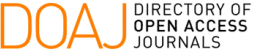### Métodos Iterativos de Regularização para Identificação do Coeficiente de Rigidez na Equação de Euler-Bernoulli para Vigas

E. F. Medeiros, A. De Cezaro, F. Travessini De Cezaro

#### Abstract

Nesta contribuição analisaremos o problema inverso de identificação do coeficiente de rigidez em vigas modeladas pela equação de Euler-Bernoulli, a partir de medidas da deflexão. Apresentaremos o problema na forma de uma equação de operador parâmetro-para-medidas, para o qual provaremos propriedades importantes, como compacidade e continuidade. Mostraremos ainda que o operador parâmetro-para-medidas é Fréchet diferenciável e que satisfaz a condição do cone tangente. Essas propriedades são suficientes para recuperarmos de forma estável e convergente (método de regularização) o coeficiente de rigidez através de métodos iterativos como Landweber e Steepest descent. Por fim,  apresentamos os efeitos de estabilidade das soluções aproximadas com relação as medidas com diferentes níveis de ruídos através de exemplos numéricos.

#### Keywords

identificação do coeficiente de rigidez; métodos iterativos; problemas inversos.

#### Full Text:

PDF (Português (Brasil))

#### References

J. GERE, Mecânica dos Materiais. Editora Cengage, 2009.

R. HIBBELER, Resistência dos Materiais. Pearson Prentice Hall, 7 ed., 2006.

A. Lerma and D. Hinestroza, “Coefficient identification in the Euler–Bernoulli equation using regularization methods,” Applied Mathematical Modelling, vol. 41, pp. 223–235, 2017.

T. MARINOV and A. VATSALA, “Inverse problem for coefficient identification in the Euler–Bernoulli equation,” Computers & Mathematics with Applications, vol. 56, no. 2, pp. 400–410, 2008.

A. KAWANO, “Uniqueness in the determination of unknown coefficients of an Euler–Bernoulli beam equation with observation in an arbitrary small interval of time,” Journal of Mathematical Analysis and Applications, vol. 452, no. 1, pp. 351–360, 2017.

S. Caddemi and A. Morassi, “Multi-cracked Euler–Bernoulli beams: Mathematical modeling and exact solutions,” International Journal of Solids and Structures, vol. 50, no. 6, pp. 944–956, 2013.

D. LESNIC, L. ELLIOTT, and D. INGHAM, “Analysis of coefficient identification problems associated to the inverse Euler-Bernoulli beam theory,” IMA journal of applied mathematics, vol. 62, no. 2, pp. 101–116, 1999.

E. LUCCHINETTI and E. STÜSSI, “Measuring the flexural rigidity in nonuniform beams using an inverse problem approach,” Inverse problems, vol. 18, no. 3, p. 837, 2002.

T. MARINOV, R. MARINOVA, and A. VATSALA, “Coefficient identification in euler-bernoulli equation from over–posed data,” Neural, Parallel, and Scientific Computations, vol. 23, pp. 193–218, 2015.

J. HADAMARD, Lectures on the Cauchy Problem in Linear Partial Differencial Equations. Yale University Press, 1 ed., 1923.

J. BAUMEISTER, Stable Solution of Inverse Problems. Vieweg, 1 ed., 1987.

A. KIRSCH, An introduction to the mathematical theory of inverse problems, vol. 120. Springer Science & Business Media, 2011.

W. GROETSCH, Inverse Problems in the Mathematical Sciences. Vieweg, 1 ed., 1993.

B. Kaltenbacher, A. Neubauer, and O. Scherzer, Iterative regularization methods for nonlinear ill-posed problems, vol. 6. Walter de Gruyter, 2008.

M. Collins and W. Kuperman, “Inverse problems in ocean acoustics,” Inverse Problems, vol. 10, no. 5, p. 1023, 1994.

E. F. Medeiros, “Identificação do coeficiente de rigidez no modelo de eulerbernoulli para vigas,” Master’s thesis, 2019.

F. GHRIB, L. LI, and P. WILBUR, “Damage identification of euler–bernoulli beams using static responses,” Journal of engineering mechanics, vol. 138, no. 5, pp. 405–415, 2011.

G. LIU and S. CHEN, “A novel technique for inverse identification of distributed stiffness factor in structures,” Journal of Sound and Vibration, vol. 254, no. 5, pp. 823–835, 2002.

H. Brezis, Functional analysis, Sobolev spaces and partial differential equations. Springer Science & Business Media, 2010.

E. KREYSZIG, Introductory Functional Analysis with Applications. Canadá, 1 ed., 1978.

M. PROTTER and H. WEINBERGER, Maximum principles in differential equations. Springer Science & Business Media, 2012.

DOI: https://doi.org/10.5540/tcam.2022.023.02.00363

### Refbacks

• There are currently no refbacks.

Trends in Computational and Applied Mathematics

A publication of the Brazilian Society of Applied and Computational Mathematics (SBMAC)

Indexed in: